Courses

# Solutions of Refraction of Light (Page No- 248) - Physics By Lakhmir Singh, Class 10 Class 10 Notes | EduRev

## Class 10 : Solutions of Refraction of Light (Page No- 248) - Physics By Lakhmir Singh, Class 10 Class 10 Notes | EduRev

The document Solutions of Refraction of Light (Page No- 248) - Physics By Lakhmir Singh, Class 10 Class 10 Notes | EduRev is a part of the Class 10 Course Class 10 Physics Solutions By Lakhmir Singh & Manjit Kaur.
All you need of Class 10 at this link: Class 10

Lakhmir Singh Physics Class 10 Solutions Page No:248

Question 41: A student did an experiment with a convex lens. He put an object at different distances 25 cm, 30 cm, 40 cm, 60 cm and 120 cm from the lens. In each case he measured the distance of the image from the lens. His results were 100 cm, 24 cm, 60 cm, 30 cm and 40 cm, respectively. Unfortunately his results are written in wrong order.
(a) Rewrite the image distances in the correct order.
(b) What would be the image distance if the object distance was 90 cm ?
(c) Which of the object distances gives the biggest image ?
(d) What is the focal length of this lens ?

Solution : (a) 100 cm; 60 cm; 40 cm; 30 cm; 24 cm
(b) When u = -25cm, v = 100cm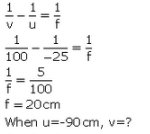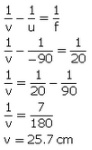(c) 25 cm
(d) 20 cm (As calculated in part (b))

Question 42: A magnifying lens has a focal length of 100 mm. An object whose size is 16 mm is placed at some distance from the lens so that an image is formed at a distance of 25 cm in front of the lens.
(a) What is the distance between the object and the lens ?
(b) Where should the object be placed if the image is to form at infinity ?

Solution :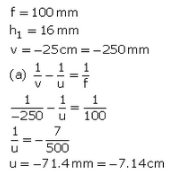Distance between object and lens Is 7.14 cm.

(b)The object shculd be placed at the focus so that the image is formed at infinity.

So, u = -100 mm = -10cm

The object should be placed 10 cm In front of the lens.

Question 43: A lens forms a real image 3 cm high of an object 1 cm high. If the separation of object and image is 15 cm, find the focal length of the lens.

Solution :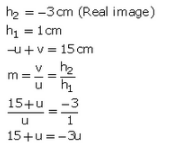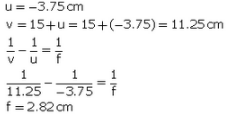Offer running on EduRev: Apply code STAYHOME200 to get INR 200 off on our premium plan EduRev Infinity!

94 docs

,

,

,

,

,

,

,

,

,

,

,

,

,

,

,

,

,

,

,

,

,

,

,

,

;